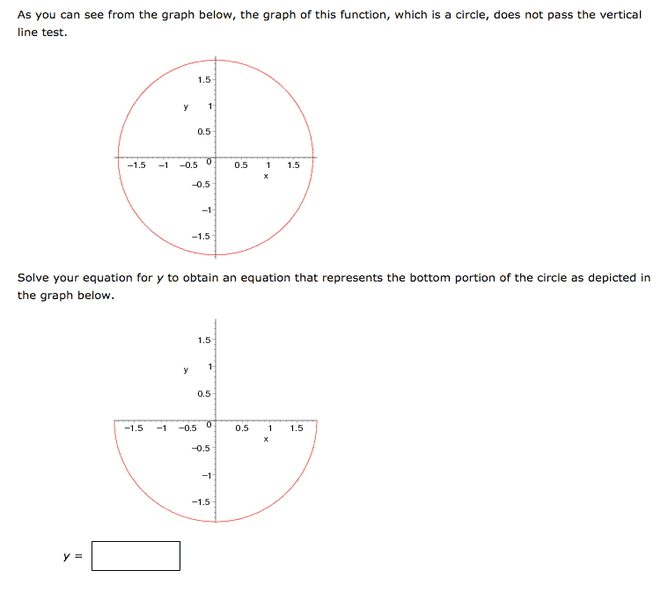# Equation for y to obtain an equation that represents circle

Niaboc67

## Homework Statement

The equation that is first listed is: 2x^2 + 2y^2 = 7

## Homework Equations[/B]

## The Attempt at a Solution

It's redefing the bottom portion therefore I think it's y=0,-2 because that is the range?

Gold Member
2021 Award

## The Attempt at a Solution

It's redefing the bottom portion therefore I think it's y=0,-2 because that is the range?
I do not get how you think that is a solution. The solution is an equation in the form of y = f(x) such that it is represented by the graph shown (the lower portion of the circle. This is trivial.